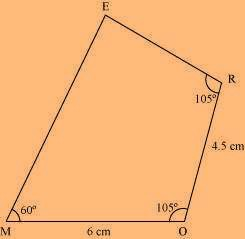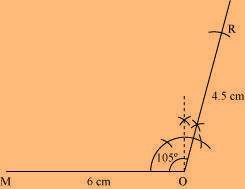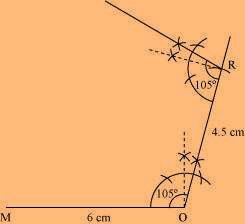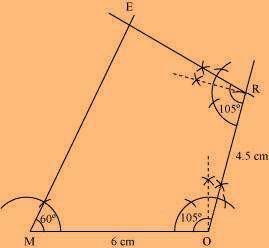# Construct the following quadrilateral: Quadrilateral MORE MO = 6 cm OR = 4.5 cm ∠M = 60° ∠O = 105° ∠R = 105°

Solution

Rough Figure:(1) Draw a line segment MO of 6 cm and an angle of 105º at point O.

As vertex R is 4.5 cm away from the vertex O, cut a line segment OR of 4.5 cm from this ray.(2) Again, draw an angle of 105º at point R.(3) Draw an angle of 60º at point M. Let this ray meet the previously drawn ray from R at point E.(0)(0)